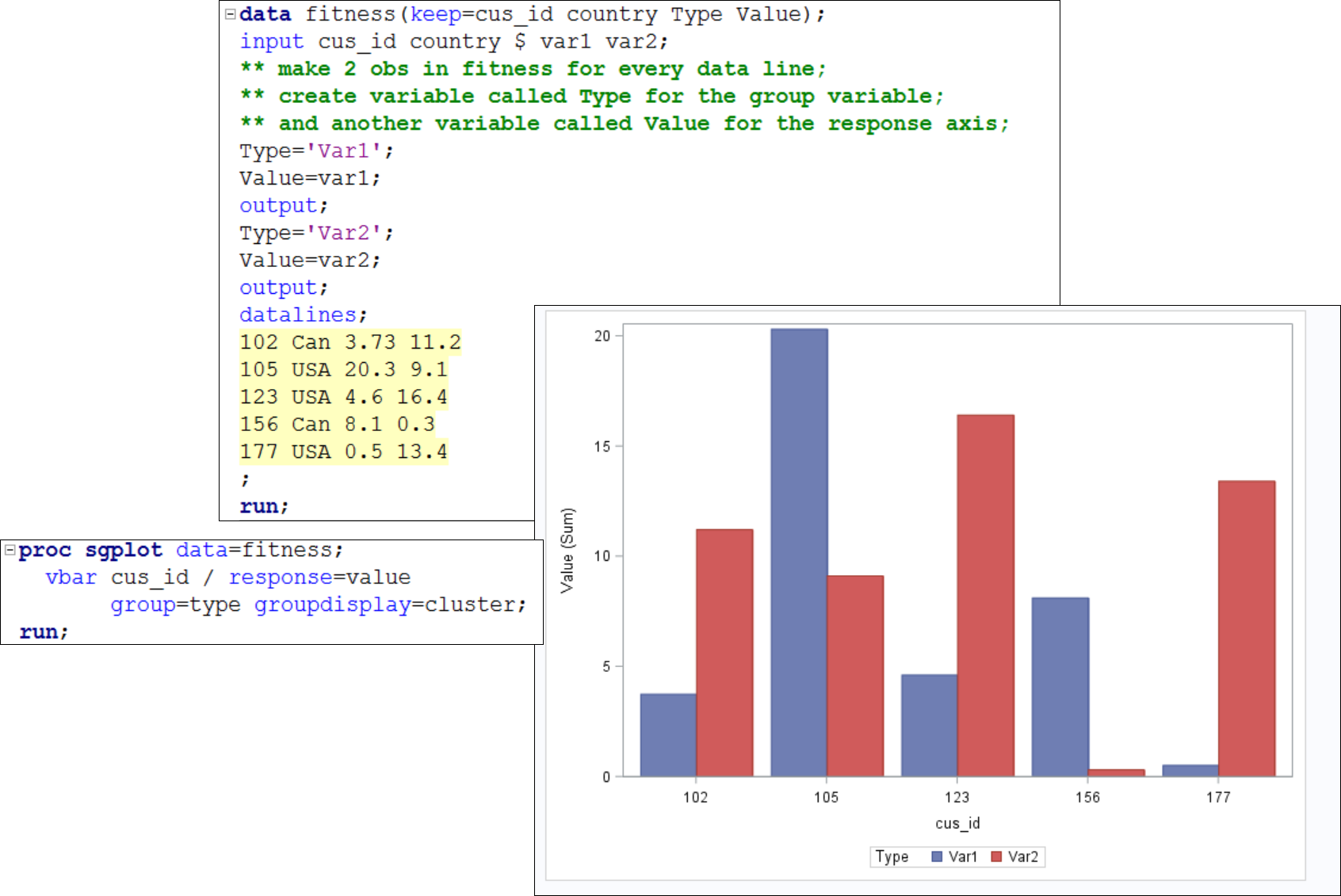## plotting two variables in one bar

Hi,

In proc sgplot with either hbar or vbar statement, how to bring information from two variables to one bar.  For eg. var1=5 and var2=10.  Other obs could be var1=2 and var2=5.  The plot should show one bar for each obs and each representaive bar should show informaion from both var1 (red) and var2 (blue).  The group won't work here.

1 ACCEPTED SOLUTION

Accepted Solutions

## Re: plotting two variables in one bar

If you want the bars stacked change GROUPDISPLAY from CLUSTER to STACK.

9 REPLIES 9

## Re: plotting two variables in one bar

Basically need to show two 'response' values into one bar with different color.

## Re: plotting two variables in one bar

You'll need to restructure you data into how SAS expects it to be for a stacked bar chart.

This means combining the variables and included a new variable to denote the source/group.

## Re: plotting two variables in one bar

You mean create a new variable by adding var1+var2.  But in the vbar statement, what will be go in response=?? and group= combined?? groupdisplay=stack?  Thanks.

## Re: plotting two variables in one bar

If you want sample code post sample data.

## Re: plotting two variables in one bar

Here is a sample data. Need to show var1 and var2 of each customer in the same bar.

data fitness;
input cus_id country \$ var1 var2;
datalines;
102 Can 3.73 11.2
105 USA 20.3 9.1
123 USA 4.6 16.4
156 Can 8.1 0.3
177 USA 0.5 13.4
;
run;

## Re: plotting two variables in one bar

Hi:

Restructuring the data will get you want you want. You just need to make 2 observations for every observation in the input data.

cynthia## Re: plotting two variables in one bar

If you want the bars stacked change GROUPDISPLAY from CLUSTER to STACK.

## Re: plotting two variables in one bar

Thanks Reeza and Cynthia..!  Did not get the restructuing part, it is clear now.

## Re: plotting two variables in one bar

The correct answer should be Cynthia's post.

Discussion stats
• 9 replies
• 4743 views
• 0 likes
• 3 in conversation